Miscellaneous

Chapter 8 Class 11 Sequences and Series
Serial order wise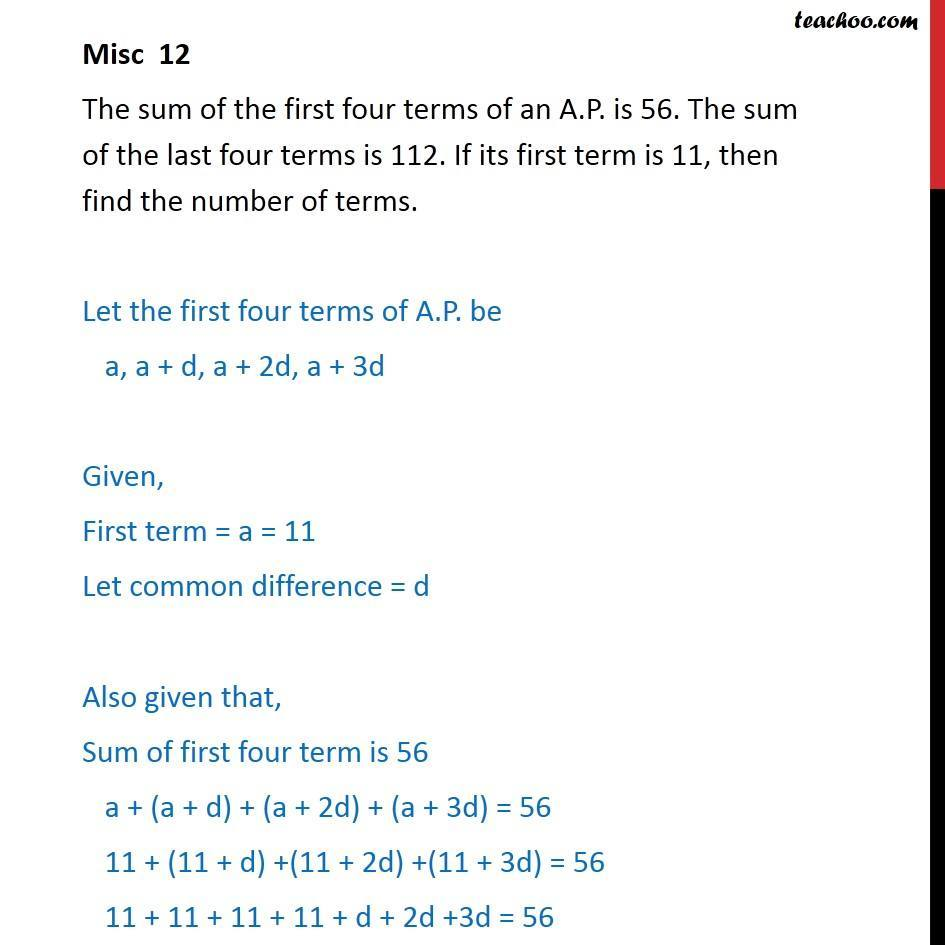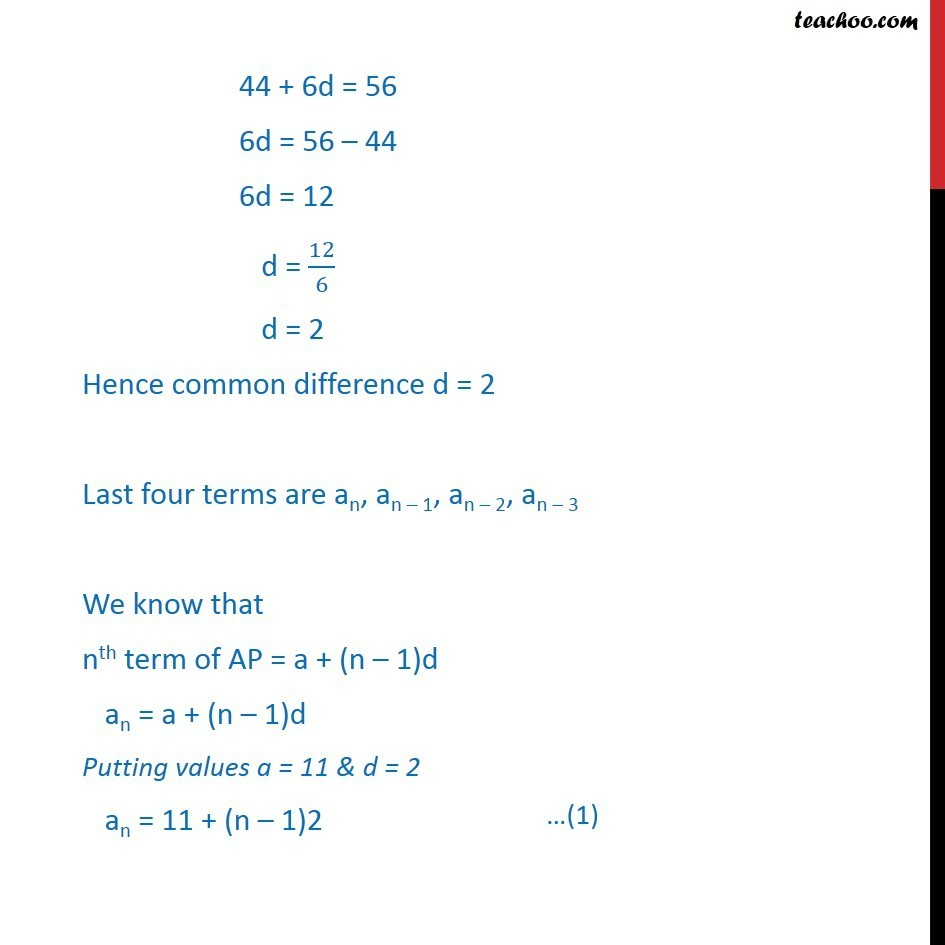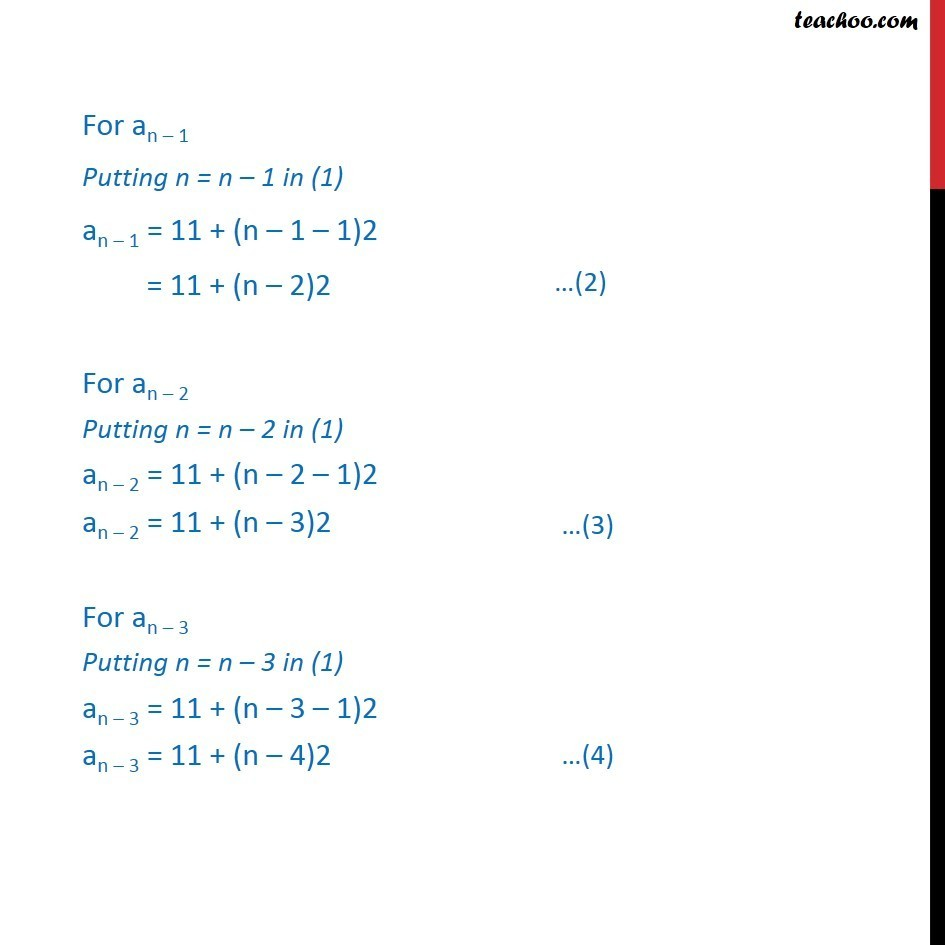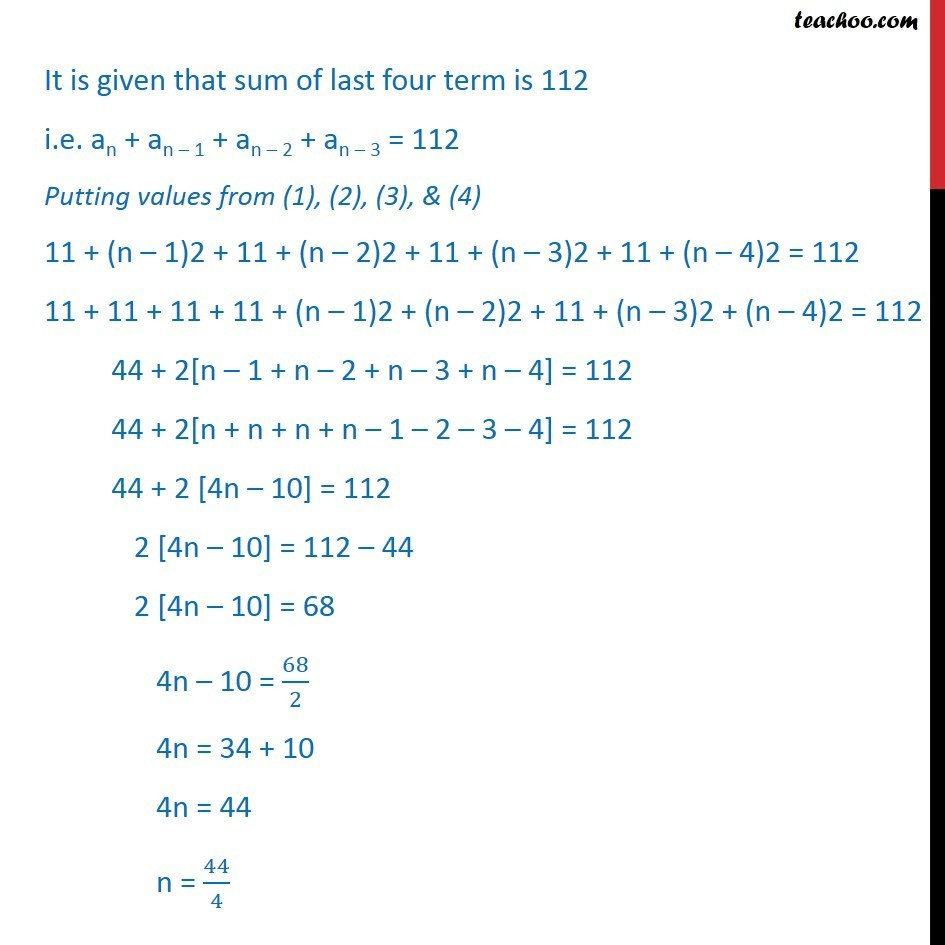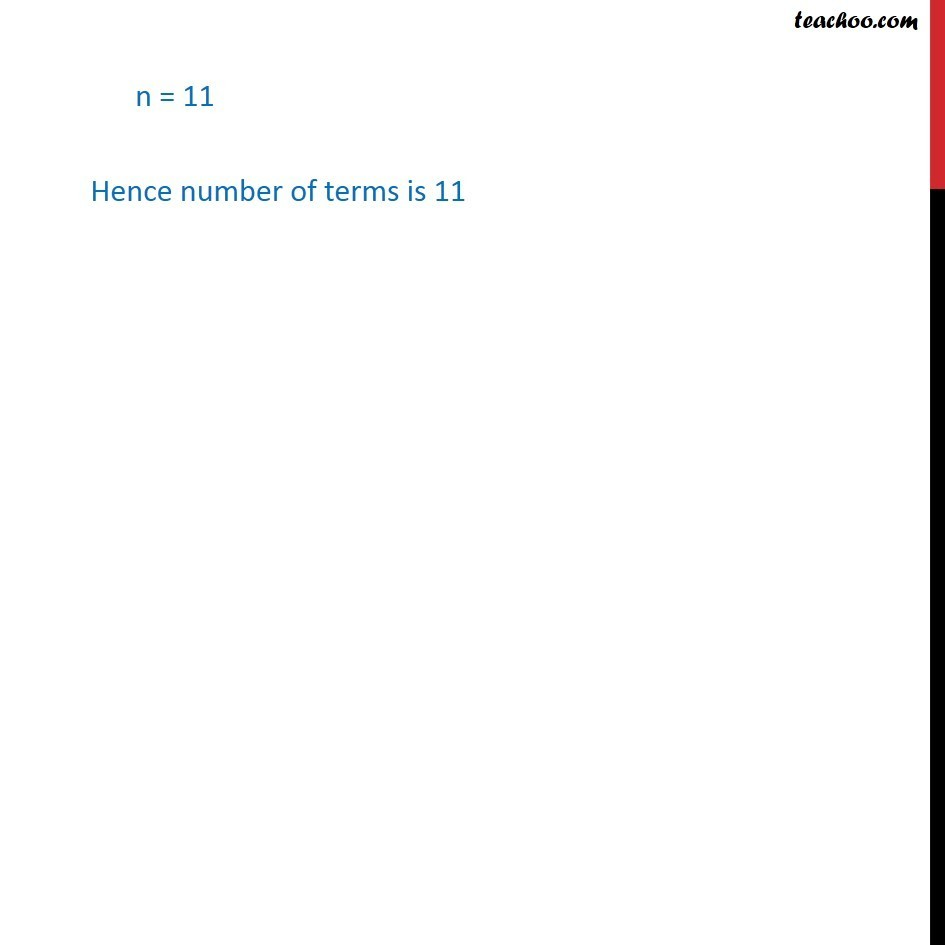Learn in your speed, with individual attention - Teachoo Maths 1-on-1 Class

### Transcript

Misc 12 The sum of the first four terms of an A.P. is 56. The sum of the last four terms is 112. If its first term is 11, then find the number of terms. Let the first four terms of A.P. be a, a + d, a + 2d, a + 3d Given, First term = a = 11 Let common difference = d Also given that, Sum of first four term is 56 a + (a + d) + (a + 2d) + (a + 3d) = 56 11 + (11 + d) +(11 + 2d) +(11 + 3d) = 56 11 + 11 + 11 + 11 + d + 2d +3d = 56 44 + 6d = 56 6d = 56 – 44 6d = 12 d = 12/6 d = 2 Hence common difference d = 2 Last four terms are an, an – 1, an – 2, an – 3 We know that nth term of AP = a + (n – 1)d an = a + (n – 1)d Putting values a = 11 & d = 2 an = 11 + (n – 1)2 For an – 1 Putting n = n – 1 in (1) an – 1 = 11 + (n – 1 – 1)2 = 11 + (n – 2)2 For an – 2 Putting n = n – 2 in (1) an – 2 = 11 + (n – 2 – 1)2 an – 2 = 11 + (n – 3)2 For an – 3 Putting n = n – 3 in (1) an – 3 = 11 + (n – 3 – 1)2 an – 3 = 11 + (n – 4)2 It is given that sum of last four term is 112 i.e. an + an – 1 + an – 2 + an – 3 = 112 Putting values from (1), (2), (3), & (4) 11 + (n – 1)2 + 11 + (n – 2)2 + 11 + (n – 3)2 + 11 + (n – 4)2 = 112 11 + 11 + 11 + 11 + (n – 1)2 + (n – 2)2 + 11 + (n – 3)2 + (n – 4)2 = 112 44 + 2[n – 1 + n – 2 + n – 3 + n – 4] = 112 44 + 2[n + n + n + n – 1 – 2 – 3 – 4] = 112 44 + 2 [4n – 10] = 112 2 [4n – 10] = 112 – 44 2 [4n – 10] = 68 4n – 10 = 68/2 4n = 34 + 10 4n = 44 n = 44/4 n = 11 Hence number of terms is 11